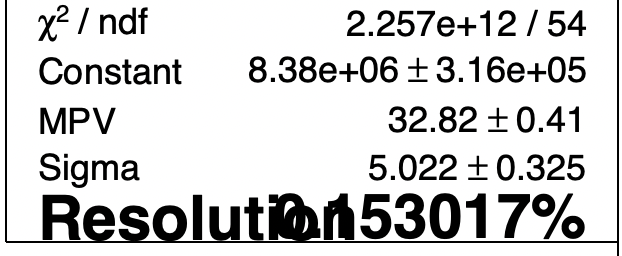# Edit TPaveStats line

I’m trying to add a line to my TPaveStats box to show the resolution of a peak (as defined by Sigma/MPV of a Landau fit). I followed the example from the class reference but it’s not working. Any help would be appreciated.

``````  TFile *_file0 = TFile::Open("myfile.root");

gStyle->SetOptStat(0);
gStyle->SetOptFit(0001);

TCanvas *c1 = new TCanvas("c1","c1",900,700);
TH1D *h1 = (TH1D*)_file0->Get("h1");
h1->Draw();
h1->Fit("landau","W","",25,60);

c1->Modified();
c1->Update();

TPaveStats *s1 = (TPaveStats*)h1->FindObject("stats");
TList *l1      = s1->GetListOfLines();

TF1 *f1 = h1->GetFunction("landau");
double MPV = f1->GetParameter("MPV");
double Sigma = f1->GetParameter("Sigma");
double Ratio = Sigma/MPV;
TString Reso = Form("Resolution = %f%%",Ratio*100);
TLatex *t1 = new TLatex(0,0,Reso);
t1 ->SetTextFont(42);
t1 ->SetTextSize(0.04);
t1 ->SetTextColor(kRed);

c1->Modified();
c1->Update();
``````

`s1->SetName("mystats"); h1->SetStats(0);`

1 Like

Great, now how can I get it to have the same formatting as the default for the other lines? Font, size, etc.

Yes I read the class reference, put more specifically I guess my question is what are the default font settings for the stats box? If I don’t set the options the new line has completely different presets, so I was wondering if instead of me going trial-and-error to get something relatively similar someone could tell me how to set it to be the same as the other linesOk I `Inspect`-ed the stats box and found that the `TextFont` was 42 and the `Size` was 0.
The final macro:

``````  TCanvas *c1 = new TCanvas("c1","c1",900,700);
TH1D *h1 = (TH1D*)_file0->Get("h1");
h1->Draw();
h1->Fit("landau","W","",25,60);

c1->Modified();
c1->Update();

TPaveStats *s1 = (TPaveStats*)h1->FindObject("stats");
s1->SetName("1");
TList *l1      = s1->GetListOfLines();

TF1 *f1 = h1->GetFunction("landau");
double MPV = f1->GetParameter("MPV");
double Sigma = f1->GetParameter("Sigma");
double Ratio = Sigma/MPV;
TString Reso = Form("Resolution = %f%%",Ratio*100);
TLatex *t1 = new TLatex(0,0,Reso);
t1 ->SetTextFont(42);
t1 ->SetTextSize(0);